EXETOOLS FORUMHex-Rays and negative structure offsets
 User Name Remember Me? Password
 Forum Rules FAQ Calendar Mark Forums Read

 Notices Any password problems please mailto: 883600(at)qq(dot)com

 Thread Tools Display Modes
#1
 jonwilVIP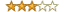Join Date: Feb 2004 Posts: 278 Rept. Given: 2 Rept. Rcvd 18 Times in 6 Posts Thanks Given: 1 Thanks Rcvd at 15 Times in 7 Posts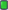Hex-Rays and negative structure offsets

I have the following structure definitions:
Code:
```#pragma pack(push, 1)
struct Vector2
{
float X;
float Y;
};
#pragma pack(pop)
#pragma pack(push, 1)
struct Vector3
{
float X;
float Y;
float Z;
};
#pragma pack(pop)
#pragma pack(push, 1)
struct VertClass
{
Vector3 Vertexes;
Vector3 Normals;
int SmGroup;
int Id;
int BoneIndexes;
int BoneWeights;
int MaterialRemapIndex;
int MaxVertColIndex;
Vector2 TexCoord;
Vector2 TexCoord2;
Vector2 TexCoord3;
Vector2 TexCoord4;
Vector3 DiffuseColor;
Vector3 SpecularColor;
Vector3 DiffuseIllumination;
float Alpha;
int VertexMaterialIndex;
Vector3 Tangent;
Vector3 Binormal;
Vector3 CrossProduct;
int Attribute0;
int Attribute1;
int SharedSmGroup;
int UniqueIndex;
int ShadeIndex;
VertClass *NextHash;
};
#pragma pack(pop)```
Then in HexRays I have this
Code:
```void __thiscall VertClass::Reset(VertClass *this)
{
int *v1; // edi
_DWORD *v2; // esi
_DWORD *v3; // eax
signed int v4; // ebp

this->Vertexes.X = 0.0;
this->Vertexes.Y = 0.0;
this->Vertexes.Z = 0.0;
this->Normals.X = 0.0;
this->Normals.Y = 0.0;
this->Normals.Z = 0.0;
this->Vertexes.X = 0.0;
this->Vertexes.Y = 0.0;
this->Vertexes.Z = 0.0;
this->Normals.X = 0.0;
this->Normals.Y = 0.0;
this->Normals.Z = 0.0;
this->SmGroup = 0;
this->Id = 0;
this->MaxVertColIndex = 0;
this->MaterialRemapIndex = 0;
v1 = this->VertexMaterialIndex;
v2 = (_DWORD *)&this->DiffuseColor.Z;
v3 = (_DWORD *)&this->TexCoord.Y;
v4 = 4;
do
{
*(v2 - 2) = 1065353216;
*(v2 - 1) = 1065353216;
*v2 = 1065353216;
v2 = 1065353216;
v2 = 1065353216;
v2 = 1065353216;
v2 = 0;
v2 = 0;
v2 = 0;
*(v1 - 4) = 1065353216;
*v1 = -1;
*(v3 - 3) = 0;
*(v3 - 2) = 0;
*(v3 - 1) = 0;
*v3 = 0;
v3 = 0;
v3 = 0;
v3 = 0;
v3 = 0;
v3 = 0;
v3 = 0;
v3 = 0;
v3 = 0;
v3 = 0;
v3 = 0;
v3 = 0;
v3 = 0;
v2 += 3;
++v1;
v3 += 16;
--v4;
}
while ( v4 );
this->BoneIndexes = 0;
this->BoneIndexes = 0;
this->BoneWeights = 100;
this->BoneWeights = 0;
this->Attribute0 = 0;
this->Attribute1 = 0;
this->UniqueIndex = 0;
this->ShadeIndex = 0;
this->NextHash = NULL;
}```
Does anyone know of a way to tell IDA that v2 and v3 point to the middle of a structure so it can print the correct structure members or any other way to make that code clearer without needing to manually calculate structure offsets?
#2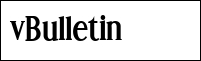GitOld Git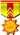Join Date: Mar 2002 Location: Torino Posts: 1,068 Rept. Given: 218 Rept. Rcvd 265 Times in 157 Posts Thanks Given: 68 Thanks Rcvd at 110 Times in 48 PostsFind your struct in Local Types and Edit it. See if it matches what you think it should be. Find you struct in Structures, open it up with ctrl-+ and you'll see all your struct members and their offsets (sorry, I'm sure you know all this). My point is - alignment. It can easily be diffent to what you think. If you are inputing your structs to IDA as a *.h file, rather than directly into Local Types, then there is an even bigger chance of the alignment beig different. I'm never sure if alignment directives in *.h files fed to IDA get interpretted or not, IDA often seems to align struct member of a 4 byte boundary for a 32 bit PE and 8 bytes for a 64bit PE.

Actually, I'm wondering... you *did* give IDA your struct definitions, yes?

Git
#3
 AvalonFriend Join Date: Jul 2018 Posts: 6 Rept. Given: 0 Rept. Rcvd 0 Times in 0 Posts Thanks Given: 1 Thanks Rcvd at 4 Times in 4 Posts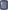Well Ida has analyzed and defined in the stack setup:
Code:
```int *v1; // edi
_DWORD *v2; // esi
_DWORD *v3; // eax```
If you create a struct of 3 PDWORD's, and apply it on the stack at v1 i.e.
Code:
```struct SomeStruct
{
PDWORD pdwField1,
PDWORD pdwField2,
PDWORD pdwField3
}```
Then Ida will remove that *v2 and *v3, and they will become struct members on the stack of SomeStruct.pdwField2 and SomeStruct.pdwField3 which you can define as any pointer type you want, with the struct you defined.
 The Following User Says Thank You to Avalon For This Useful Post: niculaita (02-20-2019)
#4
 computerlineFriend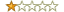Join Date: Jun 2014 Posts: 54 Rept. Given: 40 Rept. Rcvd 26 Times in 10 Posts Thanks Given: 84 Thanks Rcvd at 64 Times in 30 PostsDid you post the orginal C source of that function, hexrays can recognize negative offset, but need to known the sharp of struct, http://www.hexblog.com/?p=63

 Thread ToolsShow Printable VersionEmail this Page Display ModesLinear ModeSwitch to Hybrid ModeSwitch to Threaded ModePosting Rules You may not post new threads You may not post replies You may not post attachments You may not edit your posts BB code is On Smilies are On [IMG] code is Off HTML code is Off Forum Rules

All times are GMT +8. The time now is 17:44.

 Aaron's homepage - Top

��ICP��05004977��Always Your Best Friend: Aaron, JMI, ahmadmansoor, ZeNiX